### Linear Equations in Two Variables - Solutions

CBSE Class 9 Mathematics

NCERT Solutions
CHAPTER 4
Linear Equations in Two Variables
(Ex. 4.1)

1. The cost of a notebook is twice the cost of a pen. Write a linear equation in two variables to represent this statement.

(Take the cost of a notebook to be Rs and that of a pen to be Rs y).

Ans. Let the cost of a notebook be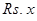.

Let the cost of a pen be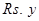.

We need to write a linear equation in two variables to represent the statement, “Cost of a notebook is twice the cost of a pen”.

Therefore, we can conclude that the required statement will be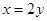= x -2y = 0

2. Express the following linear equations in the form ax by = 0 and indicate the values of aand in each case:

(i)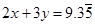(ii)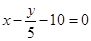(iii)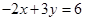(iv)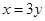(v)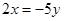(vi)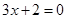(vii)(viii)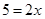Ans. (i)We need to express the linear equationin the form ax by = 0 and indicate the values of aand c.can also be written as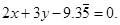We need to compare the equation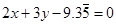with the general equation ax by = 0, to get the values of aand c.

Therefore, we can conclude that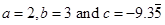.

(ii)We need to express the linear equationin the form ax by = 0 and indicate the values of aand c.can also be written as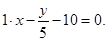We need to compare the equation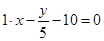with the general equation ax by = 0, to get the values of aand c.

Therefore, we can conclude that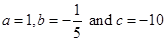.

(iii)We need to express the linear equationin the form ax by = 0 and indicate the values of aand c.can also be written as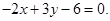We need to compare the equation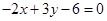with the general equation ax by = 0, to get the values of aand c.

Therefore, we can conclude that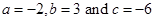.

(iv)We need to express the linear equationin the form ax by = 0 and indicate the values of aand c.can also be written as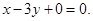We need to compare the equation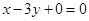with the general equation ax by = 0, to get the values of aand c.

Therefore, we can conclude that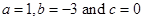.

(v)We need to express the linear equationin the form ax by = 0 and indicate the values of aand c.can also be written as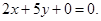We need to compare the equation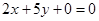with the general equation ax by = 0, to get the values of aand c.

Therefore, we can conclude that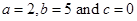.

(vi)We need to express the linear equationin the form ax by = 0 and indicate the values of aand c.can also be written as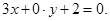We need to compare the equation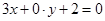with the general equation ax by = 0, to get the values of aand c.

Therefore, we can conclude that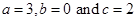.

(vii)We need to express the linear equationin the form ax by = 0 and indicate the values of aand c.can also be written as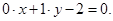We need to compare the equation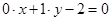with the general equation ax by = 0, to get the values of aand c.

Therefore, we can conclude that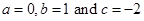.

(viii)We need to express the linear equationin the form ax by = 0 and indicate the values of aand c.can also be written as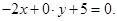We need to compare the equation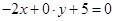with the general equation ax by = 0, to get the values of aand c.

Therefore, we can conclude that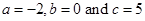.